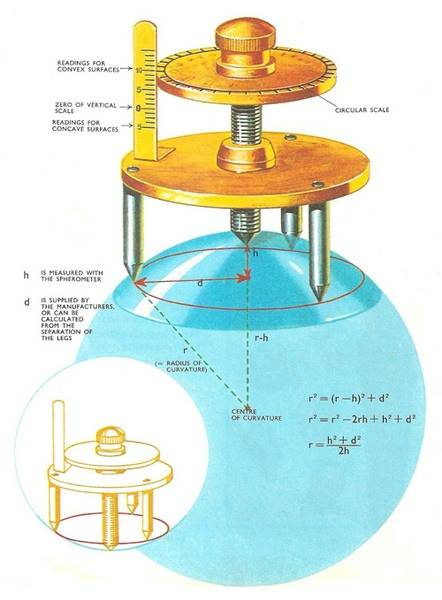# Radius of curvature using spherometer

• leojun
In summary, the conversation is about two different formulas for finding the radius of curvature using a spherometer. The first formula is correct, but the second formula is often used instead. "a" in the second formula is the same as "b" or "d" in the first formula, depending on the source. The two formulas are equivalent, but "a" in the second formula represents the side length of a triangle or the distance between two points.

#### leojun

which formula is correct?orleojun said:
which formula is correct?
The one of the picture looks correctly derived. Where does the other one come from, and what is "a"?

A.T. said:
The one of the picture looks correctly derived. Where does the other one come from, and what is "a"?

We use second formula instead of the first formula to find the radius of curvature using spherometer.
"a" in the second formula is the same as "b" in the first formula.
The first formula is correct but i don't get why we use second formula instead of first.
The two formula's are supposed to be the same r=R,but why are they different?

leojun said:
"a" in the second formula is the same as "b" in the first formula.
I see no "b" in the first formula.

A.T. said:
I see no "b" in the first formula.
SORRY,"a" in the second formula is the same as "d" in the first formula

A.T. said:
what is "a"?
"a" is the side length of the triangle, or distance between two of the three points.

•leojun
leojun said:
SORRY,"a" in the second formula is the same as "d" in the first formula
I don't think so. I think Bystander is right and the formulas are equivalent.

•leojun

## What is a spherometer?

A spherometer is a scientific instrument used to measure the radius of curvature of a spherical surface. It consists of a central stem with three or more arms extending from it, each with a small spherical foot at the end.

## How does a spherometer work?

A spherometer works by measuring the distance between the three or more feet on its arms when placed on a curved surface. By measuring this distance, along with the known distance between the central stem and the feet, the radius of curvature of the surface can be calculated using a mathematical formula.

## What is the purpose of measuring the radius of curvature?

Measuring the radius of curvature is important in various scientific fields such as optics, astronomy, and materials science. It allows researchers to determine the shape and quality of curved surfaces, which can affect the performance and accuracy of instruments and devices.

## What is the unit of measurement for radius of curvature?

The unit of measurement for radius of curvature is typically meters (m) or millimeters (mm), depending on the size of the surface being measured. In some cases, the unit of curvature may also be expressed in diopters (D), which is a unit of measurement for the refractive power of lenses.

## Is a spherometer the only way to measure radius of curvature?

No, there are other methods for measuring radius of curvature such as using a radius gauge, a spherometer attachment for a micrometer, or a profilometer. However, a spherometer is a commonly used and accurate instrument for measuring the radius of curvature of curved surfaces.